# C Program to find HCF and LCM

In this tutorial you will learn about the C Program to find HCF and LCM and its application with practical example.

## C Program to find HCF and LCM

In this tutorial, we will learn to create a C program that will find HCF and LCM  using C programming.

## Prerequisites

Before starting with this tutorial we assume that you are best aware of the following C programming topics:

• Operators in C Programming.
• Basic Input and Output function in C Programming.
• Basic C programming.
• While loop in C programming.
• Arithmetic operations in C Programming.

## Convert Number into Word

In c programming, it is possible to take numerical input from the user and find HCF and LCM with the help of a very small amount of code. The C language has many types of header libraries which has supported function in them with the help of these files the programming is easy.

With the help of this program, we can find HCF and LCM.

Here,

LCM:-Least Common Multiple.

HCF:- Highest Common Factor.

## Output:-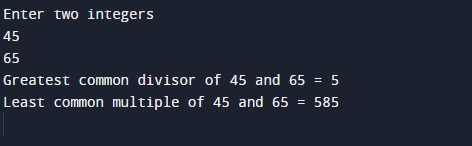The above program we have first initialize the required variable.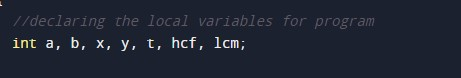• x = it will hold the integer value1.
• y = it will hold the integer value2.
• a = it will hold the integer value of x.
• b = it will hold the integer value of y.
• t = it will hold the integer temporary value.
• hcf = it will hold the integer value  of calculated hcf.
• lcm= it will hold the integer value of calculated lcm .

Input number from user.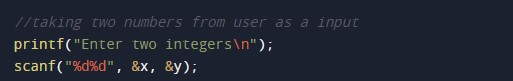Program Logic Code.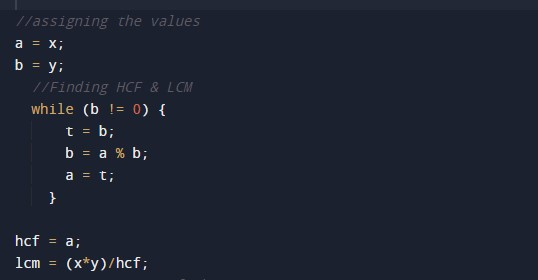Printing output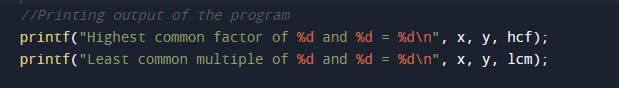In this tutorial we have learn about the C Program to find HCF and LCM and its application with practical example. I hope you will like this tutorial.